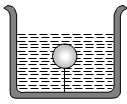The fraction of a floating object of volume ${\mathrm{V}}_{0}$ and density ${\mathrm{d}}_{0}$ above the surface of a liquid of density d will be

(a) $\frac{{\mathrm{d}}_{0}}{\mathrm{d}}$                                       (b) $\frac{{\mathrm{dd}}_{0}}{\mathrm{d}+{\mathrm{d}}_{0}}$

(c) $\frac{\mathrm{d}-{\mathrm{d}}_{0}}{\mathrm{d}}$                                   (d) $\frac{{\mathrm{dd}}_{0}}{\mathrm{d}-{\mathrm{d}}_{0}}$

Concept Questions :-

Archimedes principle
High Yielding Test Series + Question Bank - NEET 2020

Difficulty Level:

Pressure applied to an enclosed fluid is transmitted undiminished to every portion of the fluid and the walls of the containing vessel. This law was first formulated by:

(a) Bernoulli                                (b) Archimedes
(c) Boyle                                     (d) Pascal

Concept Questions :-

Pressure
High Yielding Test Series + Question Bank - NEET 2020

Difficulty Level:

A body is just floating on the surface of a liquid. The density of the body is same as that of the liquid. The body is slightly pushed down. What will happen to the body ?
(a) It will slowly come back to its earlier position

(b) It will remain submerged, where it is left

(c) It will sink

(d) It will come out violently

Concept Questions :-

Archimedes principle
High Yielding Test Series + Question Bank - NEET 2020

Difficulty Level:

A cork is submerged in water by a spring attached to the bottom of a bowl. When the bowl is kept in an elevator moving with acceleration downwards, the length of spring

(a) Increases                                          (b) Decreases
(c) Remains unchanged                           (d) None of these

Concept Questions :-

Archimedes principle
High Yielding Test Series + Question Bank - NEET 2020

Difficulty Level:

A solid sphere of density $\mathrm{\eta }$ ( > 1) times lighter than water is suspended in a water tank by a string tied to its base as shown in fig. If the mass of the sphere is m then the tension in the string is given by(a) $\left(\frac{\mathrm{\eta }-1}{\mathrm{\eta }}\right)\mathrm{mg}$                              (b) $\mathrm{\eta mg}$

(c) $\frac{mg}{\mathrm{\eta }}$                                        (d) $\left(\mathrm{\eta }-1\right)\mathrm{mg}$

Concept Questions :-

Archimedes principle
High Yielding Test Series + Question Bank - NEET 2020

Difficulty Level:

A hollow sphere of volume V is floating on water surface with half immersed in it. What should be the minimum volume of water poured inside the sphere so that the sphere now sinks into the water ?
(a) V/2                        (b) V/3

(c) V/4                        (d) V

Concept Questions :-

Archimedes principle
High Yielding Test Series + Question Bank - NEET 2020

Difficulty Level:

A ball whose density is  falls into water from a height of 9 cm . To what depth does the ball sink
(a) 9 cm                                    (b) 6 cm
(c) 4.5 cm                                 (d) 2.25 cm

Concept Questions :-

Archimedes principle
High Yielding Test Series + Question Bank - NEET 2020

Difficulty Level:

Two solids A and B float in water. It is observed that A floats with $\frac{1}{2}$ of its body immersed in water and B floats with $\frac{1}{4}$ of its volume above the water level. The ratio of the density of A to that of B is
1. 4 : 3                                  2. 2 : 3
3. 3 : 4                                  4. 1 : 2

Concept Questions :-

Archimedes principle
High Yielding Test Series + Question Bank - NEET 2020

Difficulty Level:

A boat carrying steel balls is floating on the surface of water in a tank. If the balls are thrown into the tank one by one, how will it affect the level of water ?

(a) It will remain unchanged

(b) It will rise

(c) It will fall

(d) First it will first rise and then fall

Concept Questions :-

Equation of continuity
High Yielding Test Series + Question Bank - NEET 2020

Difficulty Level:

Two pieces of metal when immersed in a liquid have equal upthrust on them; then

(a) Both pieces must have equal weights

(b) Both pieces must have equal densities

(c) Both pieces must have equal volumes

(d) Both are floating to the same depth

Concept Questions :-

Archimedes principle